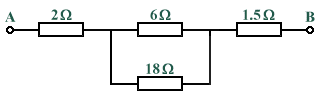# Answers to Problems - Resistor Networks

1. The voltages dropped across three resistors (R1, R2 and R3) connected in series are 5 V, 7 V and 10 V respectively. The supply current is 2 A. Calculate the supply voltage and the values of the three resistors.

VSUPPLY  =  5 V  +  7 V  +  10 V  =  22 V

 R1  = 5 V =  2.5 Ω 2 A

 R2  = 7 V =  3.5 Ω 2 A

 R3  = 10 V =  5 Ω 2 A

2. For the circuit shown below, given that the voltage dropped across R2 is 5 V and that the voltage dropped across R3 is 3 V, determine the value of the voltage dropped across R1. If the total circuit resistance is 36 Ω, calculate the supply current and the resistor values.VR1  =  18 V  -  (5 V  +  3 V)  =  10 V

 ISUPPLY  = 18 V =  0.5 A 36 Ω

 R1  = 10 V =  20 Ω 0.5 A

 R2  = 5 V =  10 Ω 0.5 A

 R3  = 3 V =  6 Ω 0.5 A

3. Two resistors are connected in series across an 18 V supply with a current of 5 A flowing through the circuit. If one of the resistors (R1) has a value of 2.4 Ω, determine the value of the other resistor (R2), and the voltage dropped across R1.

 RTOTAL  = 18 V =  3.6 Ω 5 A

R2  =  3.6 Ω  -  2.4 Ω  =  1.2 Ω

VR1  =  2.4 Ω  ×  5 A  =  12 V

4. Imagine that you have a 100 Ω resistor (R1). You want to add a resistor (R2) in series with R1 in order to limit the current to 0.5 amps when 110 volts is placed across the two resistors in series. How much resistance should you use?

 RTOTAL  = 110 V =  220 Ω 0.5 A

R2  =  220 Ω  -  120 Ω  =  120 Ω

5. Two resistors (R1 and R2) of value 4 Ω and 12 Ω are connected in parallel across a 9 V battery. Calculate the resistance of the parallel branch, the supply current, and the current flowing through each resistor.

 RTOTAL  = 4 Ω  ×  12 Ω =  3 Ω 4 Ω  +  12 Ω

 ISUPPLY  = 9 V =  3 A 3 Ω

 IR1  = 9 V =  2.5 A 4 Ω

 IR2  = 9 V =  0.75 A 12 Ω

6. Find the total resistance between terminals A and B of the circuit of the circuit shown below.RPARALLEL  = 6 Ω  ×  18 Ω =  4.5 Ω 6 Ω  +  18 Ω

RTOTAL  =  2 Ω  +  4.5 Ω  +  1.5 Ω  =  8 Ω

7. What is the equivalent resistance for this resistor combination?REQUIVALENT  = 20 Ω  ×  14 Ω =  8.235 Ω 20 Ω  +  14 Ω

8. Resistors of value 20 Ω, 20 Ω and 30 Ω are connected in parallel. Determine the value of the series resistance that must be added to the parallel combination to obtain a total resistance of 10 Ω. If the completed circuit dissipates a power of 0.36 kW, find the current flowing.

RPARALLEL  =   1  =  7.5 Ω
 1 + 1 + 1 20 Ω 20 Ω 30 Ω

RSERIES  =  10 Ω  -  7.5 Ω  =  2.5 Ω   (Additional series resistance)

I  =  √(360 W  ÷  10 Ω)  =  6 A   (Power = I 2 × R)